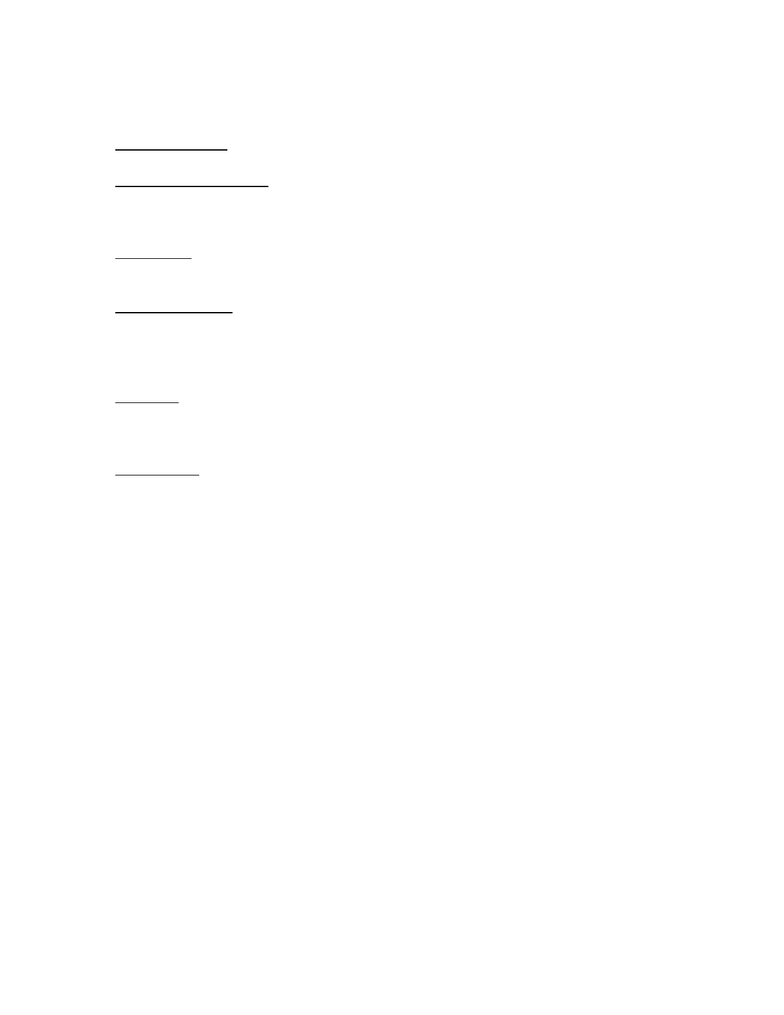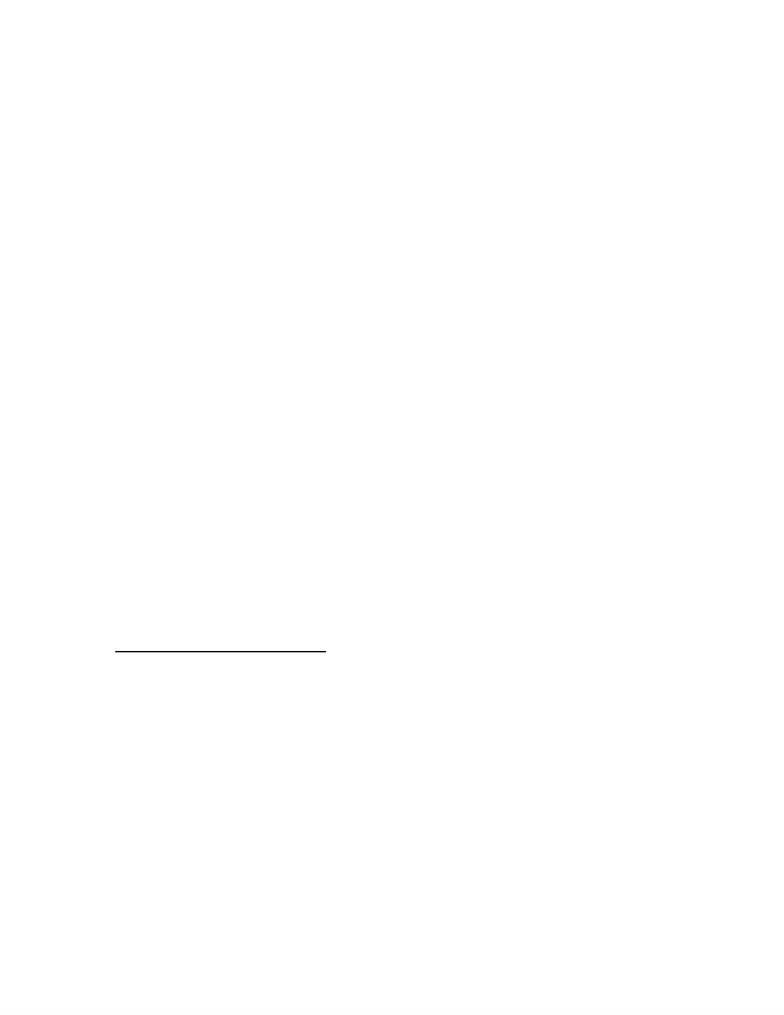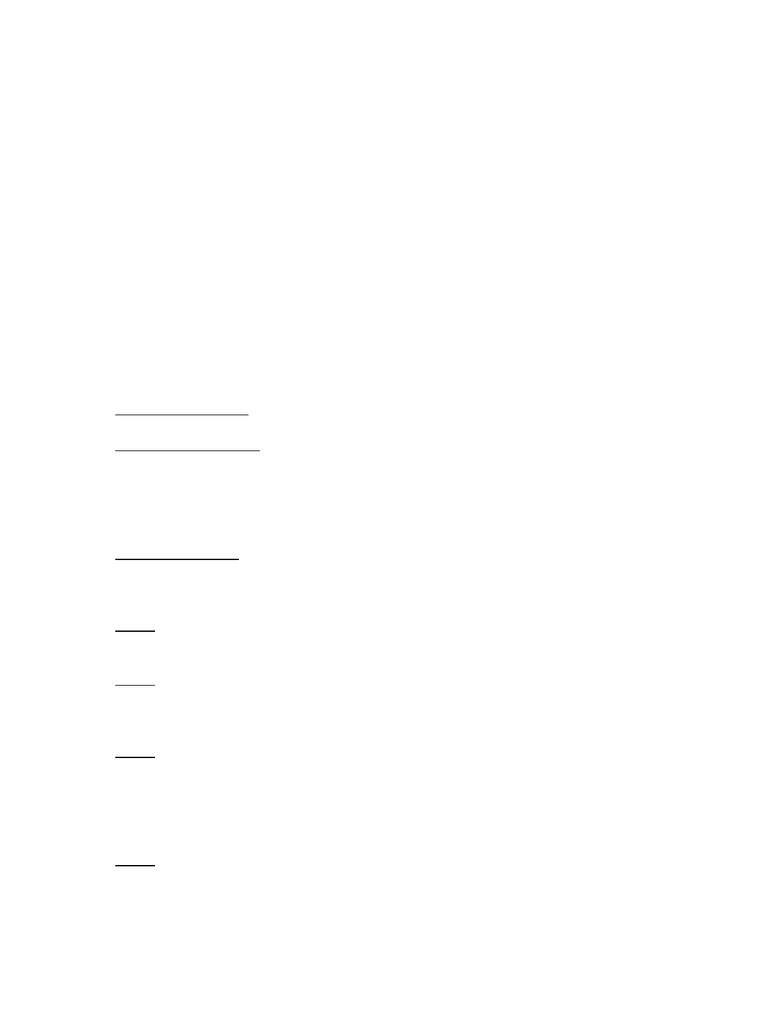# COMM 112 Lecture Notes - Cost Driver, Fixed Cost, Variable Cost

98 views14 pages
School
Department
CourseCOMM 112 Notes
Chapter 1 & 2
Managerial accounting: is the provision of accounting information for a company’s
internal users (internal focused, no mandatory rules, subjective information
possible, emphasis on the future0
Cost object: any item such as a product, customer, department, project, geographic
region, plant and so on, for which a cost are measured and assigned
Cost classification a coast is a payment of cash or the commitment to pay cash ion
the future for the purpose of generating revenues
Direct costs are those coasts that can be easily and accurately traced to a cost
object
Fixed cost is a cost that does not increase in total as output increase and does not
decrease in total as output decreases
Indirect cost are costs that cannot be easily and accurately traced to a cost object
Variable cost is a cost that does not increase in total as output increase and does not
decreases in total as output decreases
Product cost are those cost, both direct and indirect or producing a product in a
manufacturing firm or of acquiring a product in a merchandising firm and preparing
it for sale
Direct materials are those material that are a part of the final product and can be
directly traced to the goods bring produced
Direct labour is the labour that can be directly traced to the goods being produced
Manufacturing overhead: all product costs other than direct materials and direct
labour are put into a category call manufacturing overhead
Note: always take into consideration the time period and use time in order to
determine the overall cost for the raw material, goods over the given accounting
period
- In addition to calculate the prime cost and conversion cost, you must take the
total cost and divide it by either overhead or labour cost in each scenario
Direct labour cost and direct materials cost is how much is cost the organization to
purchase the raw materials and how much labour is needed to create the product
Unlock document

This preview shows pages 1-3 of the document.
Unlock all 14 pages and 3 million more documents.The overhead cost is the cost other than the direct materials and direct labour cost
that are incurred in the manufacturing process
The statement of cost of goods manufactured is prepared using the following step:
Step 1 determine the cost of direct material used
Step 2- Determine the total manufacturing costs incurred
Step 3 Determine the cost of goods manufactured
The cost of finished goods available for sale is determined by adding the beginning
finished goods inventory to the cost of goods manufactured during the period. The
cost of goods sold is determined by subtracting the ending finished goods inventory
from the cost of finished goods available for sale
Beginning inventory of materials + purchases Direct material used in production
= Ending inventory of materials
Total product cost = direct mat. + Direct labour + manufacturing OH
Unit product cost = total product cost / number of units
Prime cost = DM + DL
Conversion cost = DL + Manufacturing OH
Chapter 3 Cost behavior
Cost behavior is the general term for describing whether a cost changes when the
level of output changes. (fixed cost do not change, variable cost change with
material cost , and # of units produced)
Cost driver: a causal measurement that causes costs to change. Identifying and
managing cost drivers helps managers better predict and control costs
Relevant range: is the range of output over which the assumed cost relationship is
valid for the normal operations of a firm. This limits the cost relationship to the
range of operations that the firm normally expects to occur.
Unlock document

This preview shows pages 1-3 of the document.
Unlock all 14 pages and 3 million more documents.* fixed costs are costs that in total are constant within the relevant range as the level
of output varies (discretionary fixed costs can be changed or avoided relatively
easily at management discretion)
Variable costs are defined as costs that in total vary in direct proportion to changes
in output within the relevant range
Total variable costs = variable rate * amount of output
Note: As a result managers will analyze aspects of FC and VC in order to determine
where the main cost drivers . this will permit them to truly determine methods in
which they will be able to be more efficient and to cut costs of production
Mixed costs: are costs that have both fixed and variable components. (must break
down this cost to the FC and the VC / unit
Total cost = total fixed cost + (variable rate * # of units produced)
Dependent variable: a variable whose value depends on the value of another
variable
Independent variable: is a variable that measures output and that explains changes
in the cost or other dependent variables
Step cost displays a constant level of cost for a range of output and then jumps to a
higher level of cost at some point, where it remains for a similar range of output
High Low method
Is a method of separating mixed costs into fixed and variable components by using
just the high low data points.
Step 1 find the high point and the low point for a given data set (high point is
defined as the point with the highest activity or output level)
Step 2 using high low points calculate the variable rate
Variable rate = (high point cost low point cost) / (high point output low point
output)
Step 3 calculate the fixed cost using the variable rate (using either high or low
point)
Fixed costs = total cost at high point (variable rate * output high point)
Fixed costs total cost at low point (variable rate *output low point)
Step 4 form the cost formula for materials handling based on the high low method
Total cost = fixed cost + (variable rate * output level)
Unlock document

This preview shows pages 1-3 of the document.
Unlock all 14 pages and 3 million more documents.